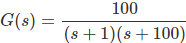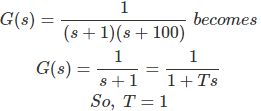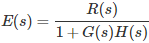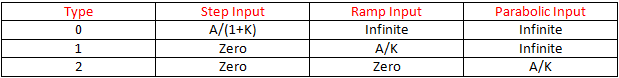# MCQs on Control Systems

##### Page 14 of 23. Go to page 1 2 3 4 5 6 7 8 9 10 11 12 13 14 15 16 17 18 19 20 21 22 23
01․ Which one of the following is the response y(t) of a casual LTI system described by H(s) = (s+1)/(s²+2s+2) for a given input x(t) = e-t u(t)?
e-t sint*u(t)
e-t cost*u(t)
e-t tant*u(t)
e-t cott*u(t)

Transfer function = Y(s)/X(s) Given input x(t) = e-t u(t) X(s) = 1/(s+1) Y(S)/X(s) = (s+1)/(s²+2s+2) = (s+1)/((s+1)²+1) Y(s) = 1/ ((s+1)²+1) By taking inverse Laplace transforms, y(t) = e-t sint*u(t)

02․ Which one of the following is the steady state error for a step input applied to a unity feedback system with the open loop transfer function G(s) = 10/(s²+14s+50)?
0
infinite
0.83
1

Error equation E(s) = R(s)/(1 + G(s) H(s) ) Applying the final value theorem, Steady state error = Lts→0 S E(s) Steady state error = Lts→0 s*1/s*1/(1+10/(s²+14s+50)) = Lts→0 1/(1+10/(s²+14s+50)) = 1/(1+10/(0+0+50)) = 1/(1+10/50) = 5/6 Steady state error = 0.833

03․ A proportional plus integral controller
has high sensitivity
increases the stability of the system
it increases rise time
both 2 and 3

Proportional plus integral controller characteristics: 1. For sinusoidal input, the phase of controller output lags by tan-1 (1/ωTi). Hence it is similar to lag compensator. 2. In terms of filtering property it acts as low pass filter. 3. It increases rise time. 4. It decreases band width. 5. It decreases stability of the system. 6.The integral controller eliminates the offset of proportional controller.

04․ Which of the following controller is also known as anticipatory controller?
proportional controller
integral controller
derivative controller
both 1 and 3

Derivative mode is also known as rate controller mode, because the controller output is proportional to rate of change of error. Its action is also known as anticipatory action. Because it sends a control signal in anticipation of error. Therefore it is also known as anticipatory controller. Anticipatory action may result in large instability in a system.

05․ Which of the following controller has sluggish response?
proportional controller
integral controller
derivative controller
both 2 and 3

For sinusoidal input the phase of integral controller output lags by 90°. Which indicates sluggish response. Therefore, the disadvantage of an integral controller is its response to errors is slow or sluggish. However, it is capable of eliminating the error completely in the system.

06․ The transfer function of a system is G(s) = 100/((s+1)(s+100)). For unit step input to the system the approximate settling time for 2% criterion is
100 sec
10 sec
400 sec
4 sec

The transfer function of a system isHere, (s + 100) is insignificant pole, so we can neglect this pole to calculate settling time.For 2% criterion, settling time ts = 4T = 4 sec.

07․ In which of the following controller band width is increased?
proportional plus integral controller
proportional plus derivative controller
both 1 and 2
nether 1 nor 2

Proportional plus derivative controller characteristics: 1. For sinusoidal input, the phase of controller output leads by tan-1 (ωTd). Hence it is similar to lead compensator. 2. In terms of filtering property it acts as high pass filter. 3. It reduces rise time. 4. It increases band width. 5. It increases stability of the system. 6. It reduces peak over shoot. 7. It has no effect on type and order of the system.

08․ Transient state analysis depends on which of the following?
type of system
order of system
both 1 and 2
nether 1 nor 2

Every transfer function representing a control system has certain type and order. The transient state analysis depends on the order of the control system. The order of system is obtained from closed loop transfer function. The highest power of the characteristic equation (1 + G(s)H(s) )determines the order of the control system.

09․ A ramp input applied to an unity feedback system results in 5% steady state error. The type number of the system is...........?
1
2
3
5

Error equationApplying final value theorem, Steady state error = Lts→0 S E(s) Steady state error for different types and different inputs as follows:10․ Time constant is defined as the time taken by the response of the system to reach ----------- ?
100% of the final value
70.7% of the final value
63% of the final value
50% of the final value

Time constant is defined as the time taken by the response of the system to reach 63% of the final value. In physics and engineering, the time constant, usually denoted by the Greek letter τ (tau), is the parameter characterizing the response to a step input of a first order, linear time invariant system. The time constant is the main characteristic unit of a first order linear time invariant system. For RC network time constant τ = RC For RL network time constant τ = L/R

<<<1213141516>>>